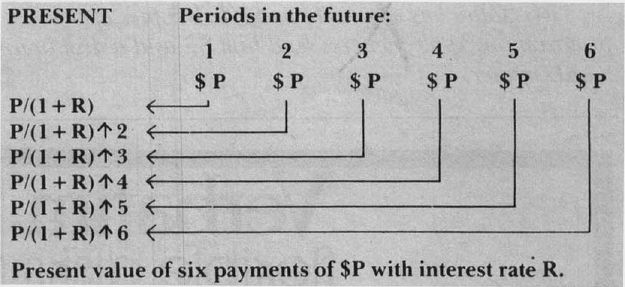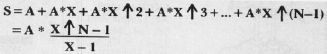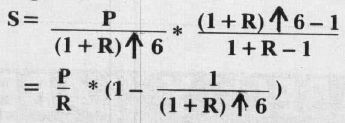`COMPUTE! ISSUE 20 / JANUARY 1982 / PAGE 48`

Keith Falkner Venice, FL

The heart of a computer program is its algorithm procedure. This is the case in this program. The purpose of the program is to solve a simple and fairly common problem in business: if a customer wishes to lease a durable article, with a view toward buying it at the end of the lease, what should the rental payment be? As written, this program limits the term to 6 or 12 or 24 or 36 months, and includes consideration of an annual charge for insurance. These considerations were part of a specific user's business environment.

The program uses an algorithm to calculate the lease payment and then verifies its result by simulating the passage of time and showing that the expected result actually happens. This will be illustrated in detail later. What is more important is how the algorithm was developed.

Creating An Interest Algorithm

Almost always, the idea behind an algorithm is very simple. This is certainly true here. The main idea is that interest is the product of principal, rate, and time. This is the simple formula which most of us have forgotten since high school.

Applying a simple formula can be a complex task, but is usually understandable in small pieces. For an example see Diagram 1, which merely illustrates that P dollars will grow to P + P * R * T dollars in T at rate R. This process can be treated in reverse: if money is to accumulate at interest in order to be worth P dollars at future time T at rate R, the present value of that money is P/(1 + R * T) dollars. These simple formulae are the heart of all interest calculations, however complicated they become.

Diagram 2 shows the values of each of six

Diagram 1payments of P dollars each, at intervals of unit time (that time which is the basis of the interest rate, e.g. 2% per month, unit time would be one month).

Diagram 2The above is simple high school math. To add up the values of the six payments, we need another idea from high school. The sum of a geometrical progression of N terms, first A, ratio X:By substituting P/(1 + R) 6 for A, and (1 + R) for X, we get:The value S above is the present value of what the customer will eventually pay in lease payments, six of them in this example. That money must equal the present value of the contract, which is the value of the article being leased, reduced by the value it will fetch after the lease is done, and increased by some fee for insurance.

Let's delve into the specific workings of the program. Table 1 identifies the variables used.

 Table 1 Variable Meaning D Fraction to buy it after lease F =1 + R(for convenience) I Annual insurance premium factor P payment each month of lease Q Optional Price to buy after lease R Rate of return as % monthly S Number of months payments T Number of the article being leased V Value of the article being leased W Worth of contract(computed) Z Insurance factor (computed)

The program collects input values for I, R, S, T, and D; since I, R, and S will usually not change, the program knows standard values for these, which should be set to your standards, not those actually shown in the listing.

Lines 140 to 170 calculate the insurance factor Z. For a six-month lease, Z is half the annual insurance factor I. For leases longer than a year, Z is I plus the present value of I for each future year of the lease.

Line 190 computes the total worth of the contract (the value of the article) plus the fee for insurance, minus the present value of the article's Eventual selling price. That present value is expressed as D*V/ F / F T. In plain English, that is the purchase-fraction D (for example. 10 to buy at 10% of original price), times V the item's value, divided by F T to bring the future selling price into the present, further divided by F, so that the customer can buy the article, not on the day of the final payment, but a month later.

At last the payment P can be computed, since W (as calculated in line 190) is equal to the sum of the series of payments calculated above as S. The payment amount P is finally calculated in line 210, and is truncated to the last cent, not rounded to the nearest cent.

The loop in line 250 simulates the behaviour of the lease as time passes. Each month the indebtedbness X is multiplied by F the interest factor, then a payment of P reduces that debt. Any debt remaining after all T payments have been made, represents the result of having ignored all the fractions of pennies which were dropped in line 230.

Well, you didn't think we were going to let the customer get away with fractions of pennies, did You? So the calculation in line 270 will show an amount slightly greater than the purchase-fraction D times the value V. Taxation laws may insist upon some minimum purchase fraction, and the above methods ensure that the final price will be at least D times V, and usually a few cents more.

The results of all this are promptly displayed on the screen. The value of the monthly payment is shown, and the eventual optional purchase price is shown, both before and after state sales tax.

Add this program to your bag of tricks, and you will have a new and potent way to attract investors. To verify that, just take a modes (nowadays) interest rate such as 2% per month, and calculate the investor's annual rate of return, which is (1 + R) 12–1. I leave to you the task of exploiting that algorithm.